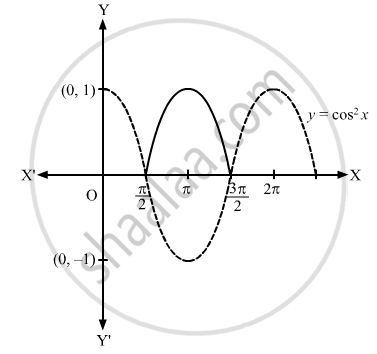Advertisement Remove all ads

# Sketch the Graph of the Following Curves on the Same Scale and the Same Axes: Y = Cos 2 X and Y = Cos X - Mathematics

Answer in Brief

Sketch the graph of the following curves on the same scale and the same axes:

$y = \cos^2 x \text{ and }y = \cos x$
Advertisement Remove all ads

#### Solution

First, we draw the graph of y = cos2 x.
Let us now draw the graph of y = cos x.
Then, we will obtain the following graph:Concept: Graphs of Trigonometric Functions
Is there an error in this question or solution?
Advertisement Remove all ads

#### APPEARS IN

RD Sharma Class 11 Mathematics Textbook
Chapter 6 Graphs of Trigonometric Functions
Exercise 6.2 | Q 2.4 | Page 8
Advertisement Remove all ads
Advertisement Remove all ads
Share
Notifications

View all notifications

Forgot password?